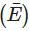Courses

# Test: Probability (CBSE Level)- 2

## 25 Questions MCQ Test Mathematics (Maths) Class 11 | Test: Probability (CBSE Level)- 2

Description
This mock test of Test: Probability (CBSE Level)- 2 for JEE helps you for every JEE entrance exam. This contains 25 Multiple Choice Questions for JEE Test: Probability (CBSE Level)- 2 (mcq) to study with solutions a complete question bank. The solved questions answers in this Test: Probability (CBSE Level)- 2 quiz give you a good mix of easy questions and tough questions. JEE students definitely take this Test: Probability (CBSE Level)- 2 exercise for a better result in the exam. You can find other Test: Probability (CBSE Level)- 2 extra questions, long questions & short questions for JEE on EduRev as well by searching above.
QUESTION: 1

### From a pack of 52 cards, the cards are drawn one by one till an ace appears. The chance that an ace does not come up in first 26 cards is

Solution:

There are 4 aces in a pack. So, the probability of drawing an ace = 4/52
= 1/13
and the probability of drawing a card other than ace = 12/13.
Hence, the probability that ace doesn't appear in first 26 draws = (12/13)26

QUESTION: 2

### Three of the 6 vertices of a regular hexagon are chosen at random.The probability that the triangle with these vertices is equilateral is

Solution:

Let T represent the equilateral triangles
n(T)= No. if equilateral triangle, with six vertices ABCDEF of regular hexagon, are only two namely △ACE;△BDF
and n(S)= Total no. of triangles with six vertices are = 6C3 = 20
P(T) = n(T)/n(S) = 2/20 = 1/10

QUESTION: 3

### In a certain town, 40% persons have brown hair, 25% have brown eyes, and 15% have both. If a person selected at random has brown hair, the chance that a person selected at random with brown hair is with brown eyes

Solution:
QUESTION: 4

The probability that a card drawn at random from a pack of 52 cards is a king or a heart is

Solution:
QUESTION: 5

Three prizes are distributed among three persons at random. The chances that none of the persons gets all the prizes is

Solution:
QUESTION: 6

The letters of the word ‘MALENKOV‘are arranged in all possible ways. The chance that there are exactly four letters between M and E is

Solution:
QUESTION: 7

The letters of the word ‘ORIENTAL‘ are arranged in all possible ways. The chance that the consonants and vowels occur alternately is

Solution:
QUESTION: 8

8 coins are tossed at a time. The probability of getting atleast 6 heads up is

Solution:
QUESTION: 9

There are 2 boxes. One box contains 3 white balls and 2 black balls. The other box contains 7 yellow balls and 3 black balls. If a box is selected at random and from it, a ball is drawn, the probability that the ball drawn is black is

Solution:
QUESTION: 10

If A and B are two mutually exclusive events, then P (A + B) is equal to

Solution:
QUESTION: 11

If E1 and E2 are two independent events, then P (E1∩E2) is equal to

Solution:
QUESTION: 12

If E is an event, then Pis equal to

Solution:
QUESTION: 13

If the probability for A to fail in an examination is 0.2 and that for B is 0.3, then the probability that either A fails or B fails is

Solution:
QUESTION: 14

A person throws successively with a pair of dice. The chance that he throws 9 before he throws 7 is

Solution:
QUESTION: 15

In a single throw of two dice, the probability of getting a total of 7 or 9 is

Solution:
QUESTION: 16

A box contains 100 tickets numbered 1, 2, 3, ….100. Two tickets are chosen at random. If it is given that the maximum number on the two chosen tickets is not more than 10, then the probability that the minimum number on them is not less than 5 is

Solution:
QUESTION: 17

Two events A and B have probabilities 0.25 and 0.50 respectively. The probability that both A and B occur simultaneously is 0.14. Then the probability that neither A nor B occurs is

Solution:
QUESTION: 18

India plays two matches each with West Indies and Australia. In any match the probabilities of India getting points 0, 1 and 2 are 0.45, 0.5 and 0.5 respectively. Assuming that the outcomes are independent, the probability of India getting atleast 7 points is

Solution:
QUESTION: 19

An unbiased dice is rolled four times. The probability that the minimum number on any toss is not less than 3 is

Solution:
QUESTION: 20

The probability that a man will live for 10 more years is 1/4 and that his wife will live 10 more years is 1/3. The probability that neither will be alive in 10 years is

Solution:
QUESTION: 21

A and B toss 3 coins. The probability that they both obtain the same number of heads is

Solution:
QUESTION: 22

Three identical dice are rolled. The probability that the same number will appear on each of them is

Solution:
QUESTION: 23

A digit is selected at random from either of the two sets {1, 2, 3, 4, 5, 6, 7, 8, 9} and {1, 2, 3, 4, 5, 6, 7, 8, 9}. What is the chance that the sum of the digits selected is 10 ?

Solution:
QUESTION: 24

If two squares are chosen at random on a chess board, the probability that they have a side common is

Solution:
QUESTION: 25

A coin tail on both sides is tossed twice. The probability of getting ‘a head’ is

Solution: